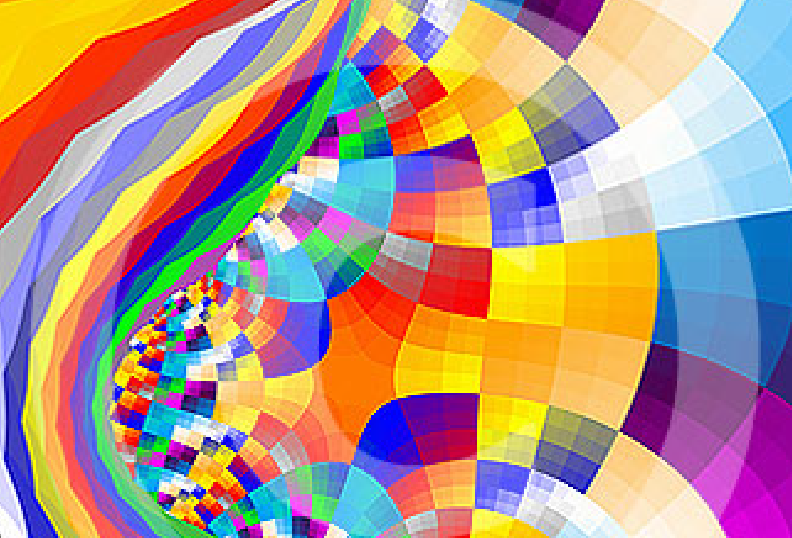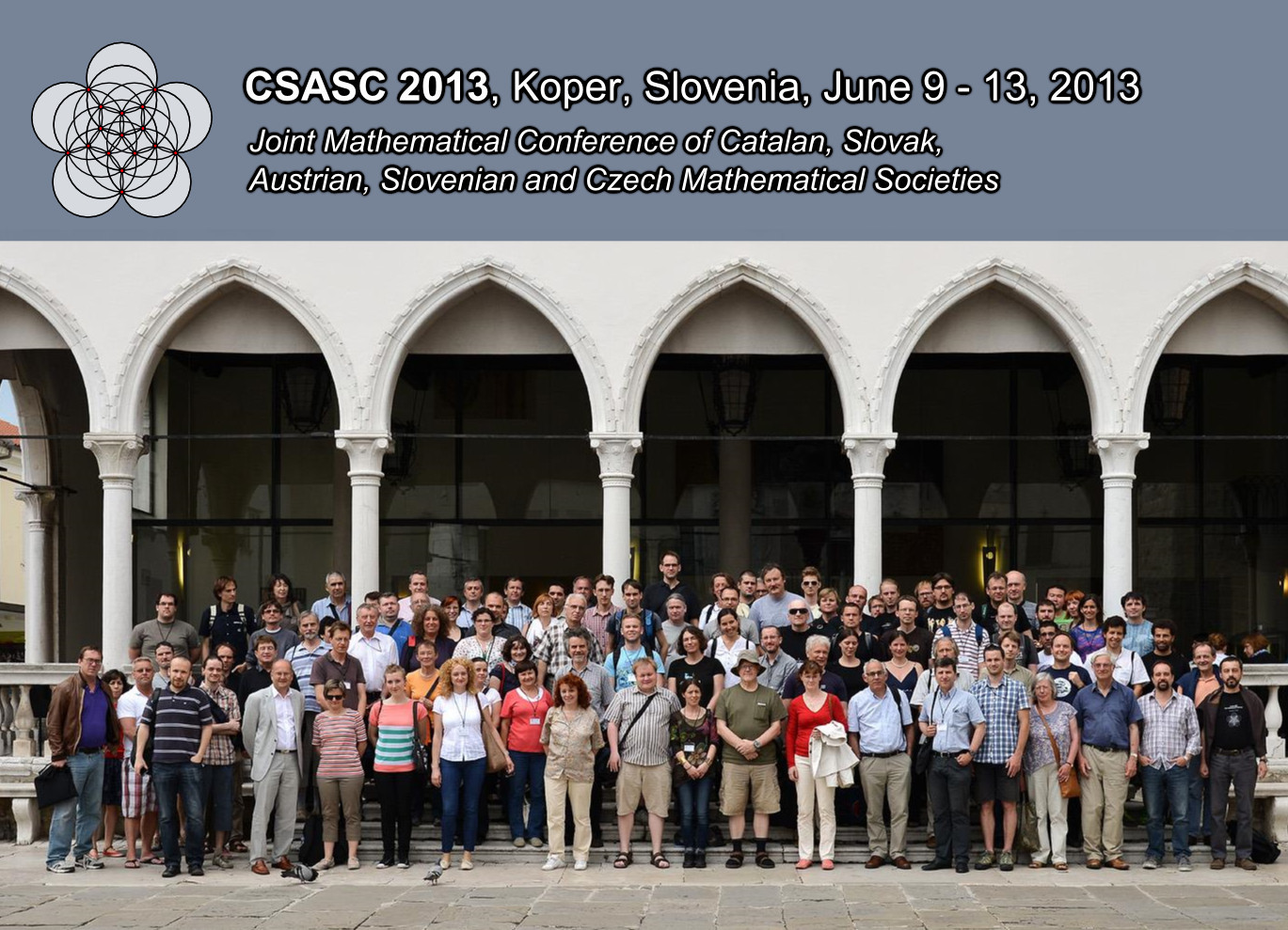University of Primorska Faculty of Mathematics, Natural Sciences and Information TechnologiesSI | EN# Mathematical Research Seminar - Archive

 2021 2020 2019 2018 2017 2016 2015 2014 2013 2012 2011 2010
 1 2 3 4 5 6 7 8 9 10 11 12
Datum in ura / Date and time: 19.4.21
(10:00 -- 11:00)
Predavalnica / Location: Zoom
Predavatelj / Lecturer: Mikhael Muzychuk (Ben-Gurion University of the Negev, Israel)
Naslov / Title: On Jordan Schemes
Vsebina / Abstract:

Motivated by the problems appearing in the theory of experimental designs, Bose and Mesner introduced in 1959 a special class of matrix algebras, known nowadays as Bose-Mesner algebras of symmetric association schemes. In the same year, Shah published the paper where he proposed a more general idea: to replace the standard matrix product with the Jordan product. So, in fact, he introduced the objects called later Jordan schemes by Cameron. While the ideas of Bose and Mesner led to a new direction in algebraic graph theory called later algebraic combinatorics by Bannai and Ito, Shah's idea was not developed at all. Only in 2004 Shah's approach was analyzed by Bailey. She observed that the symmetrization of any association scheme is a Jordan scheme, which led to the following question posed by Cameron: "Are there any others?". In my talk, I'm going to present some constructions of Jordan schemes that provide an affirmative answer to Cameron's question.

This is joint work with M. Klin and Sven Reichard.

We are looking forward to meeting at the video-conference.

Join Zoom Meeting HERE!

For more info visit our YouTube Channel.

Datum in ura / Date and time: 12.4.21
(10:00 -- 11:00)
Predavalnica / Location: Zoom
Predavatelj / Lecturer: Kenny Štorgel (UP FAMNIT and UNM FIS)
Naslov / Title: {$\ell$-Facial Edge-Coloring of Plane Graphs}
Vsebina / Abstract:

A cyclic coloring of a plane graph is a vertex coloring in which all vertices incident with the same face receive distinct colors.
It is conjectured that every plane graph with maximum face size $\Delta^*$ admits a cyclic edge-coloring with at most $\lfloor 3\Delta^*/2 \rfloor$ colors. Since in the case of plane triangulations this coloring corresponds to a proper coloring, the Four Color Theorem implies the conjecture for $\Delta^* = 3$.

As a generalization of cyclic coloring, a $k$-facial coloring was introduced, where vertices at facial distance at most $k$ receive distinct colors. An open conjecture states that every plane graph admits a $k$-facial coloring with $3k + 1$ colors.

Similarly, an $\ell$-facial edge-coloring of a plane graph is a coloring of its edges such that any two edges at distance at most $\ell$ on a boundary walk of any face receive distinct colors.
One can see that this is a more restricted version of the $k$-facial coloring by taking the medial graph $M(G)$ of a plane graph $G$.

However, there exist graphs that require $3\ell + 1$ colors for an $\ell$-facial edge-coloring. Therefore it is also conjectured that $3\ell + 1$ colors suffice for an $\ell$-facial edge-coloring of any plane graph.
While the cases with $\ell = 1$ and $\ell = 2$ are already established, the general case is still wide open.
In our talk, we give a short history of the topic and present a proof of the case $\ell = 3$.\\

This is a joint work with Borut Lu\v{z}ar.

We are looking forward to meeting at the video-conference. Join Zoom Meeting HERE!

Everyone is welcome and encouraged to attend.